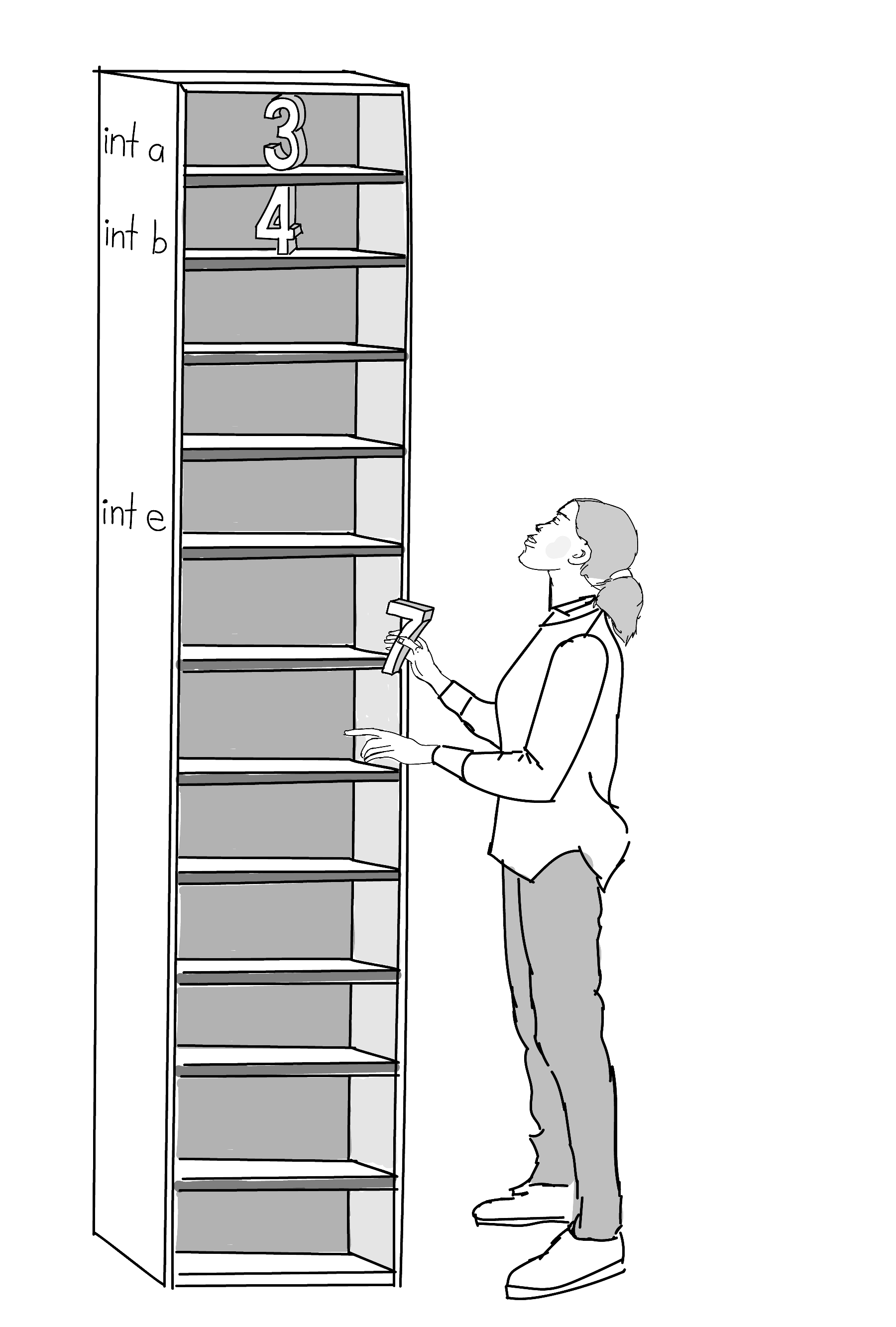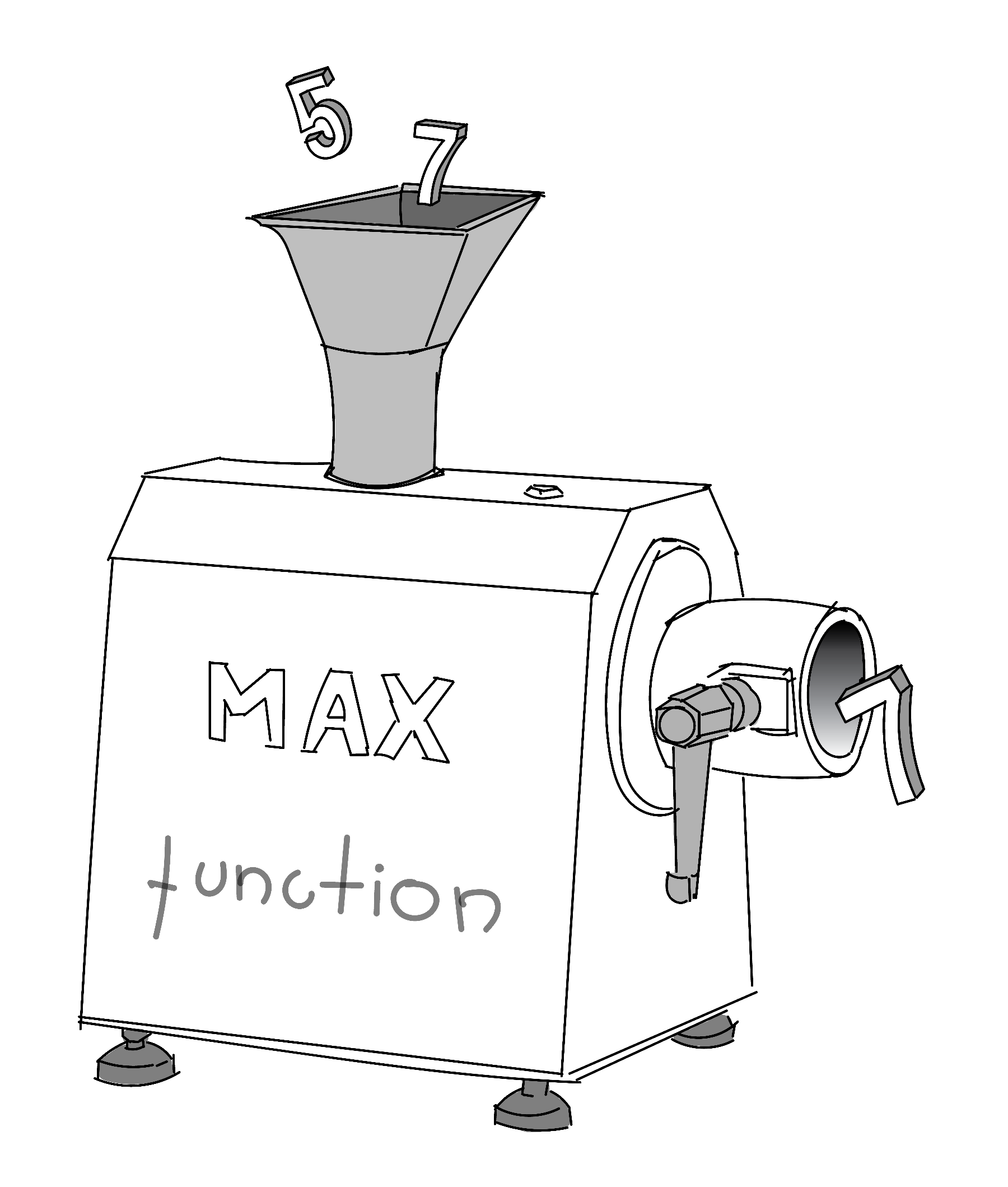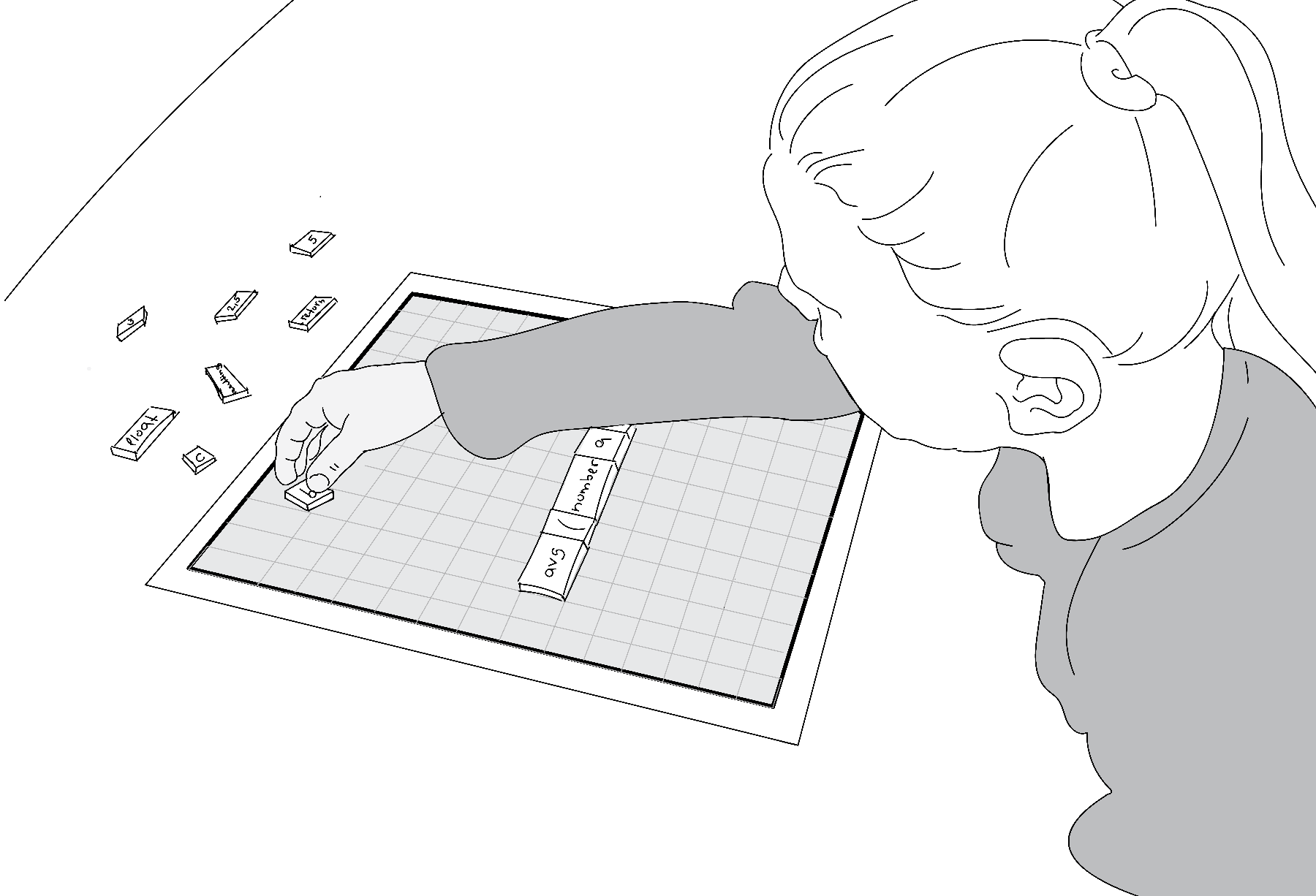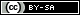# Coding Building Blocks

This page will introduce you to many of the typical building blocks programmers use to write code. If this is your first time thinking about computer code, it would be helpful to also consult the Basic Structure of a Program lesson, which is a mini-reference on the SpinWheel’s programming style.

Writing a computer program of your own, whether a desktop app, an interactive website, or the hidden brain of a robot, starts by writing a sequence of instructions in one of the many available computer languages. In this lesson, we will use a very popular older language that runs well on simple computers like the SpinWheel’s microcontroller. The name of this language is C++. While C++ has a specific set of commands and rules used to write computer programs, the main ideas are common to other programming languages. In fact, the vast majority of computer languages share the same patterns, just like how many human languages share ideas such as the distinction between a noun and a verb, or the difference between a word and a sentence. We will describe the most important such patterns in this chapter.

## Variables

Computer programs do one thing and one thing only: process information. That information can be the time of a mouse click, a voicemail on your phone, or a picture of the road taken by a self-driving car. Before processing such data, we have to tell the computer to store it in its memory. This is done using variables.“Variables” are like labeled shelves for information. When you need to save a number for later use, you put it in a variable of your choice. Above we have number 3 stored in the variable a, number 4 stored in variable b and the number 7 is about to be stored in a variable named e. We can pick the names for the variables ourselves (any sequence of letters that is not interrupted by whitespaces). image credit Mariya Krastanova

We will only discuss two types of variables: integers and decimals. Other types do exist, but we won’t cover them here. If you store a number as an integer, it must be a whole number (like 2 or 3011) and cannot have a decimal component. On the other hand, a decimal can have a decimal part (like 0.4, 560.17, or 2.0). Integers are easier for a computer to work with because it does not need to store all of the data after the decimal point. Treating them separately from decimals lets us have faster code, which is especially important for small computers that don’t have much storage space like in the SpinWheel.

To define a new integer variable you need the following line in your code:

int my_special_integer = 6;

This reserves a location in the memory of the computer, lets us refer to that location by the name my_special_integer, and stores the value 6 there. We can name the variable anything as long as it is a single word (or multiple words separated with underscores). We usually pick names that tell us something about the purpose of the variable. In this variable type, we can store any integer we want as long as it is not too large (no larger than roughly 30 000, due to limitations of how this computer stores integers).

If we want to work with decimals, we use the variable type float instead of int. The name comes from the early history of computers and is unimportant for our purposes (how the decimal point can “float” between the digits).

float pi = 3.1415;

Here we stored an approximation of the number $$\pi$$ in a variable with the name pi. We could then use this variable in other parts of our code to do computations that involve circles.

Notice that throughout all of our code we have used the equality sign = to mean “store the value on the right in the memory cell on the left”. This differs from the usual mathematical meaning of the sign, which usually means “check if the left and right side have the same value”. You can blame early computer scientists and their laziness for the misuse of this sign in most modern programming languages.

Now that you’ve learned more about variables, we encourage you to look at the start of Arduino 101 that discusses variables again. As you move through the online material and deepen your understanding of the SpinWheel, we encourage you to go back and forth between the various online pages.

## Functions

In computer programming, functions are commands that take a few variables and do something useful with them. Functions are reusable pieces of code. A function can act like a calculator, computing a new value based on the variables that are given to it. A function can also do something that affects the world around it, like blinking an LED, playing a sound, or sending a message.

Most programing languages have some functions built into them, similar to how a new cellphone comes with pre-installed apps. We can use these functions without having to write them ourselves. Here is some code that uses an example function called max that takes two numbers as input and returns the larger number. The input values are also called the arguments of the function.

int number_a = 5;
int number_b = 7;
int resulting_number = max(number_a, number_b);

Let’s step through each part of this code.

• int number_a = 5 assigns the value of 5 to the integer variable number_a
• int number_b = 7 assigns the value of 7 to the integer variable number_b
• int resulting_number = max(....) store the result of the function max(...) in the integer variable resulting_number
• max(number_a, number_b) calls, or uses, the function max(...) with two arguments, number_a and number_b, and returns the larger number.

The value stored in resulting_number in this case would be 7.Functions are tools provided in a given programming language that are capable of ingesting a number of parameters and producting (a.k.a “returning”) some new value that depended on the input parameters. image credit Mariya Krastanova

Here is another example where one of the arguments for our function is specified directly, without first being stored in a variable. In this case, the value stored in resulting_number will be 8:

int my_number = 6;
int resulting_number = max(my_number, 8);

As you have seen, the typical syntax rules for the use of a function are to put its arguments inside parentheses immediately after the name of the function. You might have seen this in math class with trigonometric functions like $$\sin{(x)}$$ or $$\cos{(x)}$$.

We can nest functions and use arithmetic operations on the arguments as well. For instance, here we will use two more functions, min which returns the smaller of two numbers and sqrt which returns the square root of a given number. Can you explain why the value stored in resulting_number in the following example is 4? Here is a hint: $$\sqrt{5-1}=\sqrt{4}=2$$.

int number_a = 5;
int number_b = 7;
int resulting_number = max(sqrt(number_a-1) * 2, min(number_b, 2));Now we have the pieces to begin to create our own functions. In the beginning, this may be like creating a poem using a limited set of magnetic word tiles. With practice, your bank of pieces will increase in size, enabling you to write more complicated code. image credit Mariya Krastanova

A large part of programming is creating your own functions and building interesting, complex, and useful functions out of small simple functions. Here we give an example of how to write your own function that takes two numbers, $$x$$ and $$y$$, and returns their average, $$\frac{x+y}{2}$$. We will name the function avg. Let us first write an example of how this function would be used if it already existed:

float x = 3.5;
float y = 2.5;
float resulting_number = avg(x, y);

In this code example, resulting_number will have the value of 3.0.

To define this new function, we need to write down its name, together with the type of data it will be producing, followed by a set of computational instructions:

float avg(float first_argument, float second_argument) {
return 0.5*(first_argument+second_argument);
}

Let’s step through each part of this code.

• float avg(.....): The very first float specifies the type of data the function will produce (in this case they are decimals). This is followed by the name we have picked for our function, avg.
• (float first_argument, float second_argument): In parentheses, we have a list of the arguments the function will be taking. Unlike when we call the function, we have to specify their types, so we wrote float to denote working with decimals. We also gave temporary names for these arguments so that we can refer to them in the function.
• { ... } : The curly brackets surround the instructions of our function.
• 0.5*(first_argument+second_argument) : This where the math happens in our function. It is simply the sum of the two arguments multiplied by one-half.
• return : a keyword to state that the result of this line of code should be returned to the code that called the function.

We can have multiple sequential instructions inside the block when the computation is more difficult. That is the purpose of the curly brackets { } - to separate all the code that defines our function from the rest of the program that might be in the same file. For instance, here we will show how to compute the fourth root of a number. The fourth root of a number, or x raised to the $$\frac{1}{4}$$ power, can be computed by taking the square root of the square root and we will use this fact to write the fourth root function below.

float root4(float x) {
float intermediate_value = sqrt(x);
return sqrt(intermediate_value);
}

Maybe the functions avg and root4 seem too redundant to you, and you would prefer to always write (x+y)/2 instead of avg(x,y). This is quite valid feeling for such short functions, but as you build more complex programs you will have longer pieces of code that would be cumbersome to repeat every time. Functions let you have a shorthand notation, so you do not need to make such repetitions.

## Functions that do not return values

Functions can also be used to change something in the environment of the device instead of being used as advanced calculators. Such functions do not return a value and don’t need a variable to store their output. One example of such a function is the delay function that pauses the computer for the length of time specified by the input variable. In the following example, calling the delay function will pause the program for 1000 milliseconds (which equals one second) before executing the next line:

a = calculation1();
delay(1000);
b = calculation2();

While this built-in function is nice, what if we want to specify the delay in seconds instead of milliseconds? When writing our own functions that do not have a return value, we specify the type of data that the function will be returning as void. This denotes that the returned value is empty or “void”. Our new function takes the number of seconds as its input, calculates the number of milliseconds corresponding to the provided number of seconds and then uses the delay function to pause the program for that length of time. We do not need to use the return keyword because our function doesn’t return a value.

void delay_seconds(int number_of_seconds) {
int number_of_milliseconds = 1000 * number_of_seconds;
delay(number_of_milliseconds);
}

As the code we write grows longer, it helps to add notes in the code to ourselves and our friends who might view our code. This makes it easier to understand the purpose of the code when we look at it again in the future. Such notes are usually called “comments”, and are completely ignored by the computer when it runs the code. In the language we are using, comments are denoted by a double slash // – everything to the right of these symbols, until the end of the line, is a message for fellow humans. We will use such comments below in some more complicated examples of code.

// I am writing this comment to remind me that the next
// line stores the number 5 in the variable named my_test_variable.
int my_test_variable = 5;

## Putting it all together

After we have created all the variables and functions we need for our code to do what we want it to do, we need to actually start the program. To do this, the program needs to know what function to run first. In different languages this is done differently. In our particular case, we do it by defining two special functions: setup and loop. Our computer is instructed to run these functions first. It finds the setup function and runs it before anything else. Usually this function is used to set up any settings we need in advance. Then the computer repeatedly runs the loop function, which is named this way because it runs in a loop (or repeats itself).

To learn more about the setup and loop functions, check out the Basic Structure of a Program lesson.

Here is a large example that includes all these features. It will use the Serial.println() function in order to send messages to the computer. Use the Serial Monitor tool in the Arduino software in order to see these messages being sent back over the USB cable (Tools → Serial Monitor).

// Here we define a convenient pausing function
// that waits a given number of seconds
void delay_seconds(int number_of_seconds) {
int number_of_milliseconds = 1000 * number_of_seconds;
delay(number_of_milliseconds);
}

// This is the setup function that will automatically run
// first when the device starts.
void setup() {
// The next line ensures that the communication hardware
// on our device is ready to send messages.
// The name "Serial" is such for historical reasons
// (it is the name for this type of communication).
Serial.begin(9600); // The 9600 is the speed of the conection.
}

// Define a variable in which we will store a counter that goes up by one on
// each step.
int counter = 1;

// This function runs repeatedly after the setup function finishes.
void loop() {
// Send a message to the connected computer.
// The message will just be the value of the counter.
Serial.println(counter);
// Increment the value of the counter.
counter = counter + 1;
// Wait for a second before you start the loop function again.
delay_seconds(1);
}

Read the comments in the code above to try to understand what each line does. One great way to test your understanding is to consider what would happen if you change the code. For example, if you changed the line counter = counter + 1 to the line below, how would the output change?

counter = counter + 2

Variables and functions are two of the most important building blocks of programming. At this point, we encourage you to continue to Intro to Animation to continue discovering more about programming!

We provide more information about the Serial Monitor in our intro to the Arduino Software page. The Arduino community also has very detailed resources on the programming language that we are using. You can start with their tutorial for instance.This work is licensed under a Creative Commons Attribution-ShareAlike 4.0 International License. © SpinWearables LLC (license and trademark details)